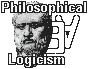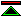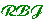# The Philosophy of Logic

## Overview:

 A philosophical approach to the understanding of logic, its significance and its applications.
 What is Logic? (philosophical) Logic is the study of necessary truths and of systematic methods for clearly expressing and rigorously demonstrating such truths. What is Logic? (symbolic) An introduction to symbolic logic. Definitions of Logic Some ways of defining "Logic" are introduced and their merits discussed.
 Necessity and Contingency We consider the notions of necessary and contingent partly through consideration of possible worlds, in order to explicate logical truth. Logicism (mathematical) Logicism is a philosophical theory about the status of mathematical truths, to wit, that they are logically necessary or analytic. Logicism (philosophical) Philosophical Logicism revisits Bertrand Russell's dream of a philosophy made logically rigorous, taking advantage of a century of development in logic, mathematics and computing.

## What is Logic (philosophical):

 Logic is the study of necessary truths and of systematic methods for clearly expressing and rigorously demonstrating such truths.
 why this definition? other definitions Necessity and Contingency To understand logic it is first necessary to grasp the important distinction between contingent propositions, which might or might not be true, and necessary propositions, which could not be false. support for the distinction against the distinction Logic and Analytic Philosophy Because the methods of analytic philosophy are mainly a priori philosophy is concerned with logic not only as a subject matter but as an important contribution to the methods of philosophy. Analytic Philosophy IS Logic Analytic Philosophy IS NOT Logic

## What is Logic? (symbolic):

 an introduction to symbolic logic
 Introduction Logic is for constructing proofs, which give us reliable confirmation of the truth of the proven proposition. Formal Languages A language is formal if the syntax of the language is defined with sufficient precision that a computer could be programmed to check whether any particular sentence belongs to the language.
 Formal Logics An inference rule is a method of deriving conclusions from premises. When inference rules for a formal language are codified it becomes a formal logic. Varieties of Logic There are many different kinds of logical system within which proofs can be conducted.
 Philosophy A bit of philosophy might help us understand logic. Or it might not. Glossary Brief explanations of some of the words which have special significance in logic.

## Definitions of Logic:

 Some ways of defining "Logic" are introduced and their merits discussed.
 Introduction A bit of preliminary discussion about what we are looking for here. Evaluation Criteria How do we know when we have a good definition? Here are some criteria which we can use to assess candidates. methods, systems, truths are all involved, a definition can be approached through any one methods Logic can be defined as concerned with methods for reasoning. Logical systems are then formalisations of the proper methods and logical truths are those demonstrable by correct methods. systems logic can be defined as concerned with a certain class of logical systems, logical truths would then be defined in terms of their derivibility in the systems. truths Here certain kinds of truths are the definitive concern, systems and methods are then judged in terms of their ability to confirm this kind of truth.

## Necessity and Contingency:

 We consider the notions of necessary and contingent partly through consideration of possible worlds, in order to explicate logical truth.
 introduction We compare three different definitions of necessity (and hence of contingency). some necessary propositions Four examplary propositions are presented, one for each kind of necessity considered, and one which is unequivocally contingent.
 defining necessity and contingency We then sketch three alternative kinds of necessity, between which the examples serve to discriminate. possible worlds Finally we consider the relationship between necessity and possible worlds, showing the conception of possible world which corresponds to each of our notions of necessity.

## Mathematical Logicism:

 Logicism is a philosophical theory about the status of mathematical truths, to wit, that they are logically necessary or analytic.
 What it is Just the claim that the theorems of mathematics are logically necessary or analytic. Some Original Readings The primary sources of the logicist doctrine are Frege and Russell. What it isn't Not a claim about formalisability, either in principle or in any specific logical system. Not a claim about how mathematical truths are discovered or applied. Not concerned any aspect of mathematics other than the theorems that mathematicians seek to demonstrate.
 Arguments Against Logicism It is generally understood that set theory is required to do modern mathematics. The existence of sets is, however, not logically necessary. The truths of mathematics are therefore contingent. Arguments For Logicism The truths of mathematics are the same in all possible worlds, since they do not depend upon the existence of sets, just upon the consistency of the supposition that the required sets exist. Since true in every possible world, mathematics must be logically necessary.

##Philosophical Logicism revisits Bertrand Russell's dream of a philosophy made logically rigorous, taking advantage of a century of development in logic, mathematics and computing.
 Introduction Philosophical logicism investigates what analytic philosophy might be like if it were exclusively concerned with logical analysis. Epistemology Logicist Epistemology is an important subtheme of philosophical logicism. Logic A broad conception of logic is needed, encompassing as much as possible of the a priori. Logic also contributes formal notations, suitable for support by intelligent software, and modern analytic methods for effective man-machine collaboration.
 Mathematics Logic, as the formal treatment of the a priori, encompasses mathematics. An adequate foundation for logic must also suffice for mathematics. The philosophy of mathematics is therefore of importance to philosophical logicism. Metaphysics Metaphysics (in a rather constrained sense) has a contribution to make to our understanding both of the nature of logical truth, and of the application of analysis in science and engineering.
 Science Science is concerned with formulating and testing general empirical theories from which specific factual conclusions can be deduced. We consider the benefits of greater formality in this process. Engineering Engineering design applies scientific models to the design of desirable artefacts. We carry through from the formalisation of science to the automation of engineering design.©created 1995-2-24 modified 1998-12-23